## Hashing

### Integer, String as Indexes

We’ve now seen several implementations of the Set (or Map) ADT.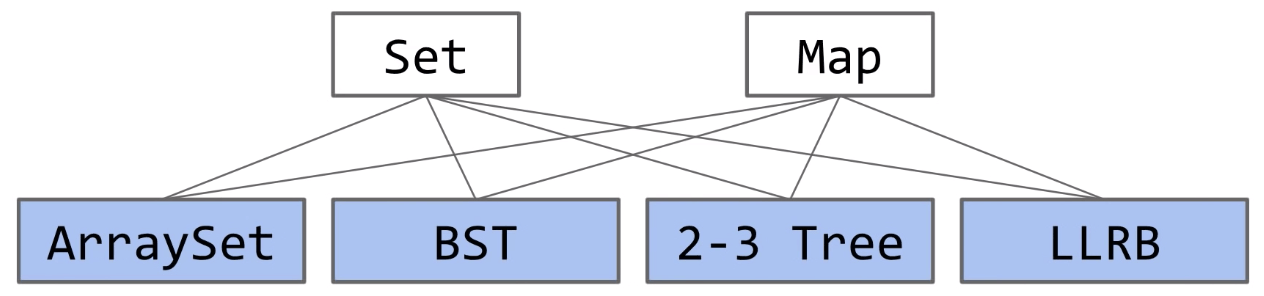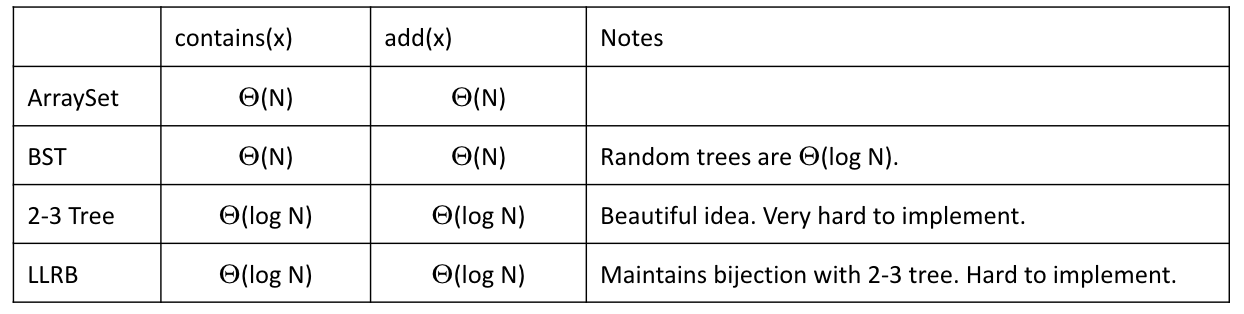Limits of Search Tree Based Sets:

• Our search tree based sets require items to be comparable.
• Our search tree based sets have excellent performance, but could maybe be better $\Theta(1)$?
• $\Theta(\log{N})$ is amazing. 1 billion items are still only height $~30$.

Using Data as an Index & Its Downsides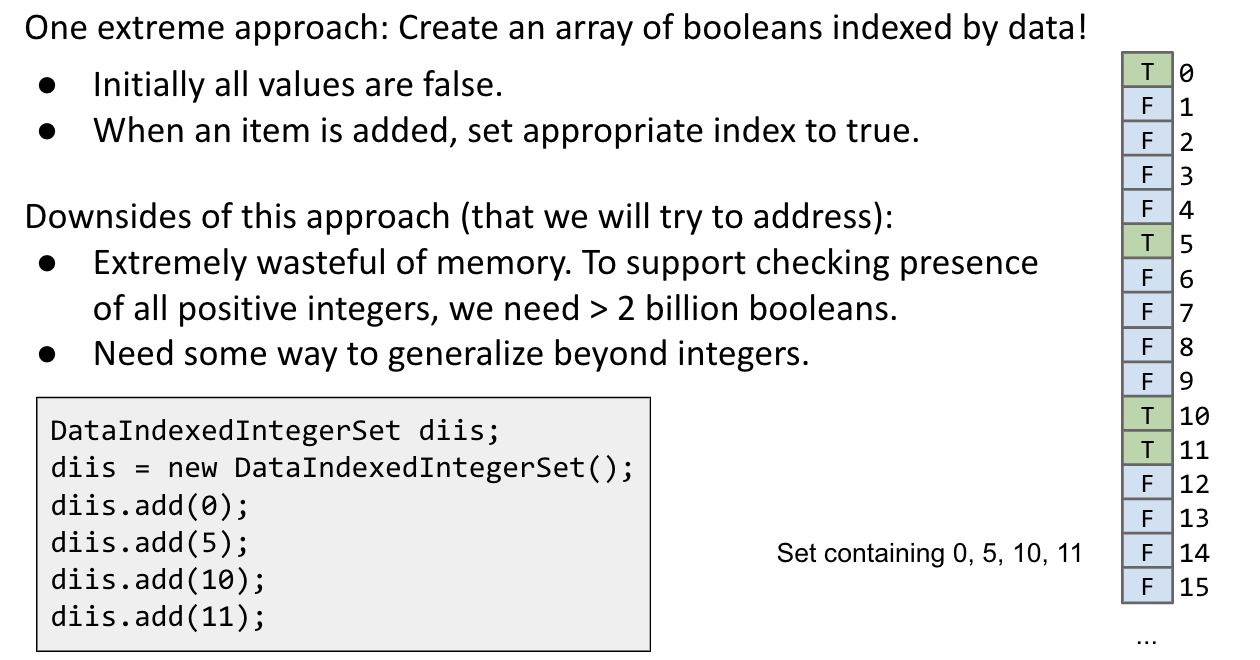Using English words as indexes and avoiding collisions (just mimicking decimal number system)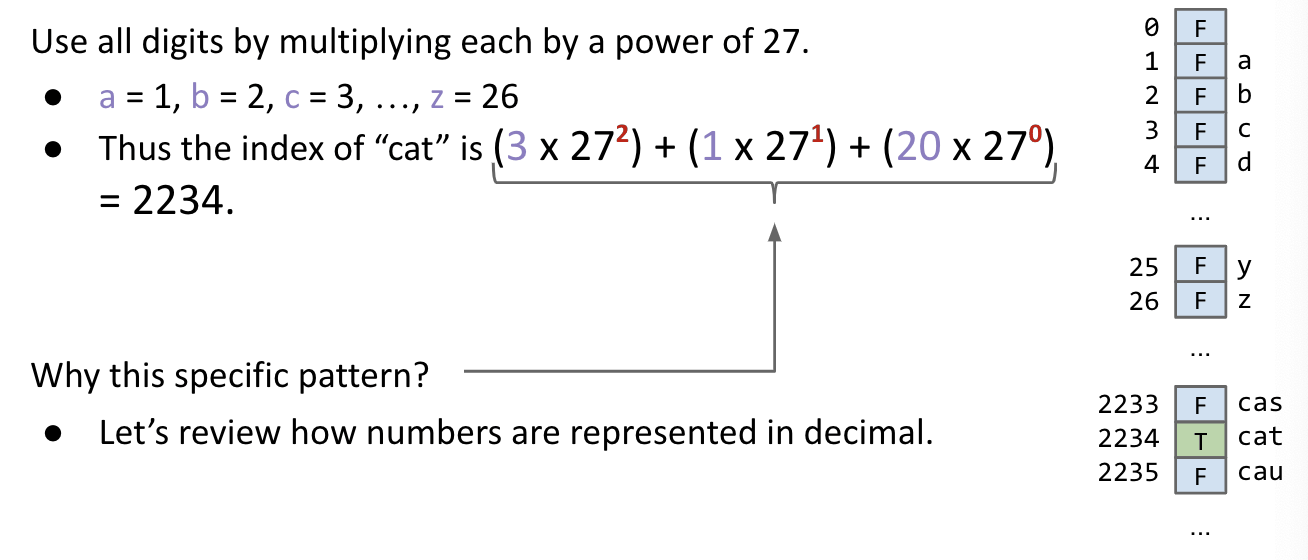It turns out as long as we pick a base >= 26, this algorithm is guaranteed to give each lowercase English word a unique number (no collision)!

Implementing letterNum:

Implementing englishToInt:

Using ASCII to represent more symbols

Implementing asciiToInt:

Consequence of Overflow: Collisions

Overflow can result in collisions, causing incorrect answers due.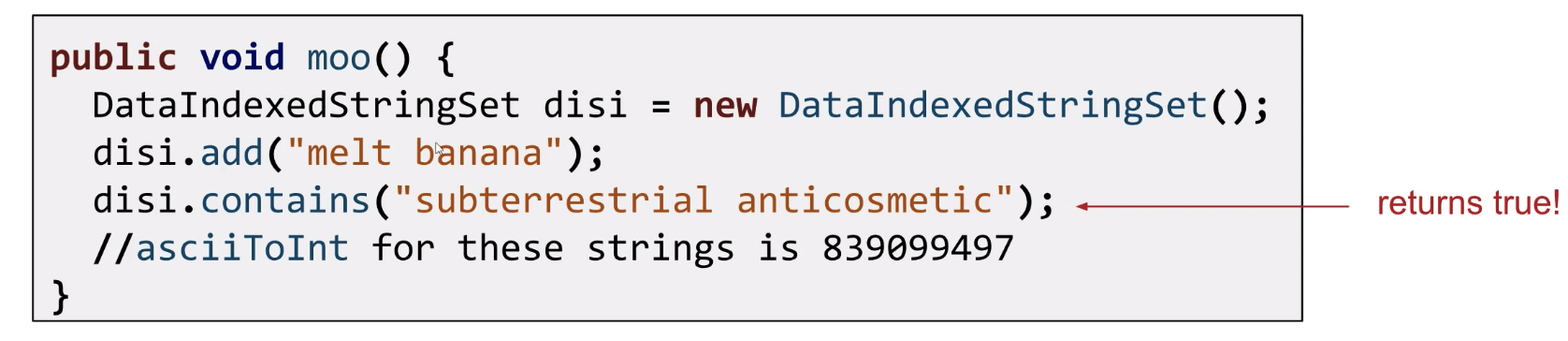### Hash Code and the Pigeonhold Principle

Via Wolfram Alpha: A hash code “projects a value from a set with many (or even an infinite number of) members to a value from a set with a fixed number of (fewer) members.”

In Java, the size of our target set is 4,294,967,296.

Pigeonhole principle tells us that if there are more than 4,294,967,296 possible items, multiple items will share the same hash code.

Bottom line: Collisions are inevitable.

Collision handling: Resolve hashCode collisions.

Computing a hashCode: How to compute a hash code for arbitrary objects?

### Hash Tables: Handling Collisions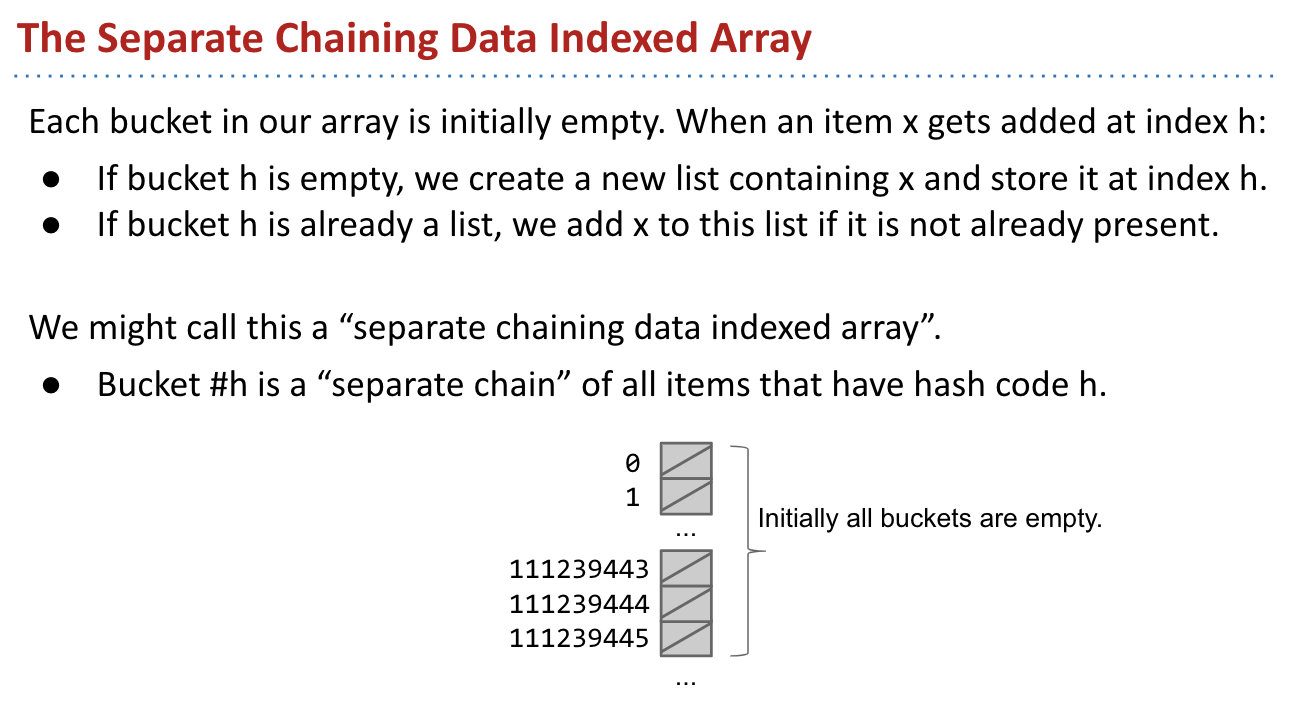Runtime: $\Theta(Q)$. (Q: Length of the longest list)

Can’t we just add to the beginning of the LinkedList, which takes $\Theta(1)$ time? No! Because we have to check to make sure the item isn’t already in the LinkedList.

• Data is converted by a hash function into an integer representation called a hash code.
• The hash code is then reduced to a valid index, usually using the modulus operator, e.g. 2348762878 % 10 = 8. (solving space)

The good news: We use way less memory and can now handle arbitrary data.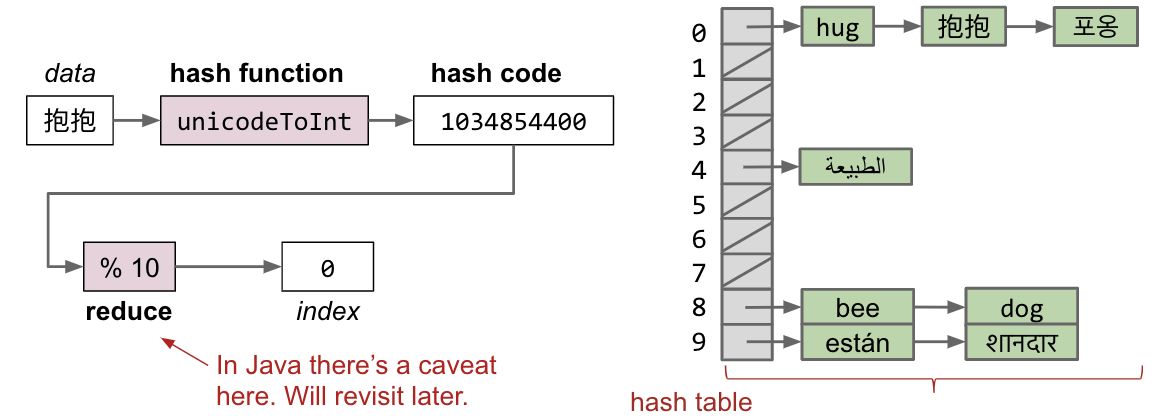In terms of $Q$, the length of the longest list will be $\frac{N}{5}$ in the best case, while it will be $N$ in the worst case. So, $Q(N)$ is $\Theta(N)$.

How can we improve our design to guarantee that $\frac{N}{M}$ is $\Theta(1)$?

• Resizing: Using an increasing number of buckets $M$. (e.g. linear to $N$)
• As long as $M = \Theta(N)$, then $O(\frac{N}{M}) = O(1)$. ($\frac{N}{M}$ is called the load factor)

E.g. When $\frac{N}{M} \geq 1.5$, then double $M$.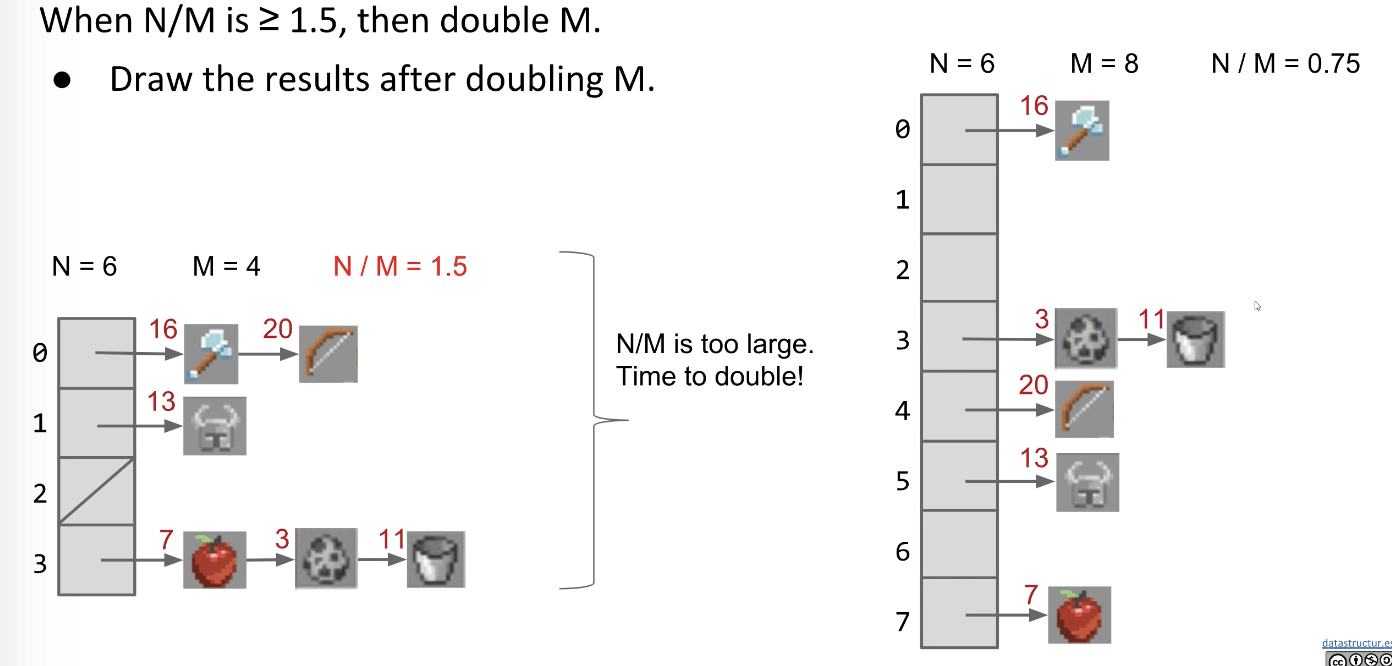Assuming items are evenly distributed, lists will be approximately $\Theta(\frac{N}{M})$ runtimes.

• Doubling strategy ensures that $\frac{N}{M} = O(1)$.
• Unless that operation causes a resize, which takes $\Theta(N)$ time.
• But similar to AList, as long as we resize by a multiplicative factor, the average runtime will still be $\Theta(1)$.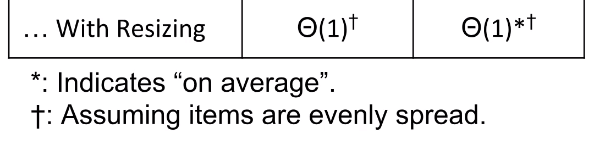Note that we need to add elements one by one again because since the size of the array changes, the modulus also changes, therefore the item probably belongs in a different bucket in the new hash table than in the old one.

A Small Point: when doing the resize, we don’t actually need to check if the items are already present in the LinkedList or not (because we know there are no duplicates), so we can just add each item in $\Theta(1)$ time for sure by adding it to the front of the linked list (addFirst). (Recall that usually we have to search the LinkedList to make sure the item isn’t there… but we can skip that step when resizing.)

### Hash Tables in Java

Hash tables are the most popular implementation for sets and maps.

• Great performance in practice.
• Don’t require items to be comparable.
• Implementations often relatively simple.
• Python dictionaries are just hash tables in disguise.

In Java, each object has a function called hashCode, whose default implementation simply returns the memory address of the object.

How do use a negative hash code?

In Java, -1 % 4 = -1, which will result in index errors! Instead, Math.floorMod(-1, 4) will return 3.

Two Important Warnings When Using HashMaps/HashSets

Warning #1: Never store objects that can change in a HashSet or HashMap!

• If an object’s variables change, then its hashCode changes. May result in items getting lost.
• For example: Set of Lists

Warning #2: Never override equals without also overriding hashCode.

• Can also lead to items getting lose and weird behavior.
• HashMaps and HashSets use equals to determine if an item exists in a particular bucket.

### Good Hash Functions

Can think of multiplying data by powers of some base as ensuring that all the data gets scrambled together into a seemingly random integer.

For example, the Java 8 hash code for strings:

• Represents strings as a base 31 number.
• Why small? Real hash codes don’t care about uniqueness.
• Stores (caches) calculated hash code so future hashCode calls are faster.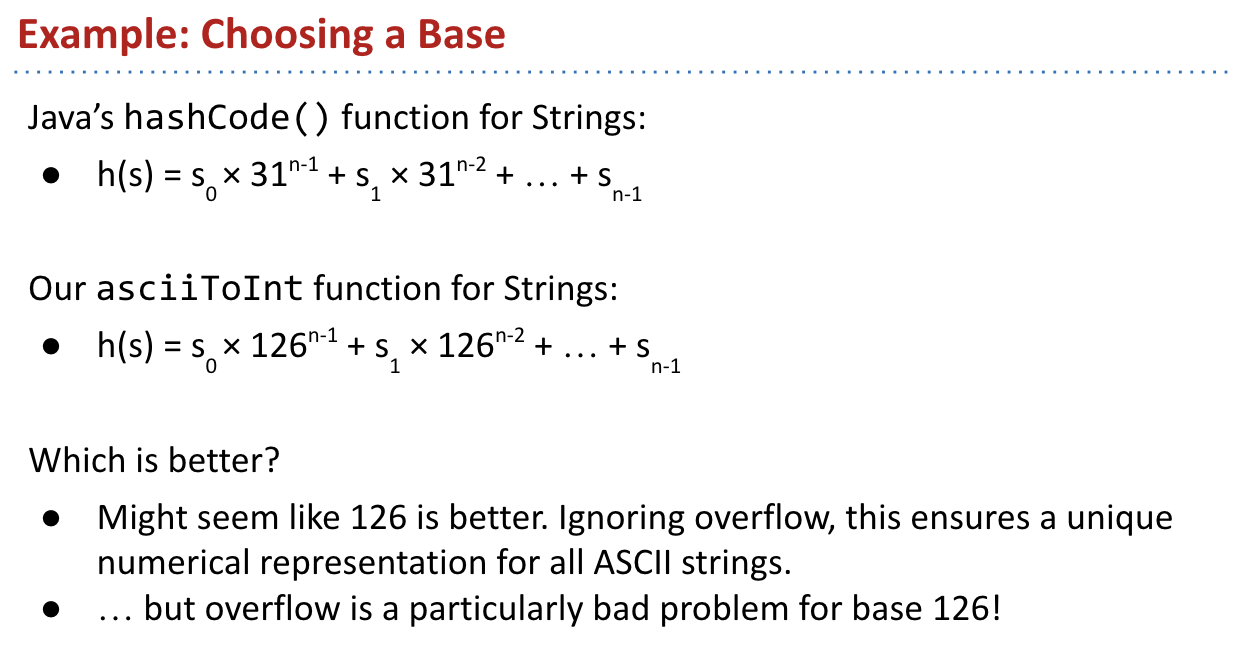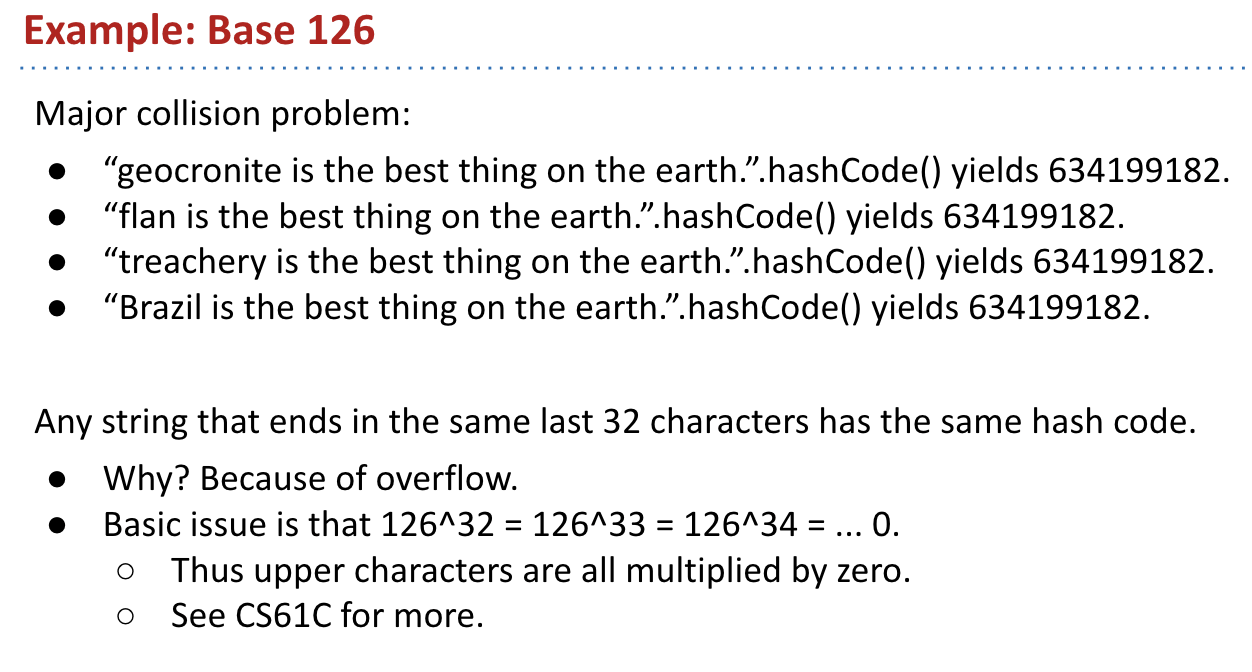Typical Base

A typical hash code base is a small prime.

Why prime?

• Never even: Avoid the overflow issue on previous slide.
• Lower chance of resulting hashCode having a bad relationship with the number of buckets.

Quora: Why are prime numbers used for constructing hash functions?

Why small?

• Lower cost to compute.

Hashing a Collection

To save time hashing: Look at only first few items. Higher chance of collision but things will still work.

Hashing a Recursive Data Structure

### Linear Probing Hash Tables

The above content is called External Chaining. Here is another solution called Linear Probing.

Linear Probing Hash Tables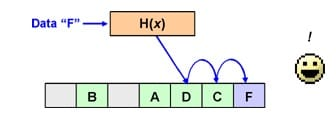## Ex (Discussion)

### Ex 1 (Valid Or Not?)

Categorize each as either a valid or an invalid hash function.

Valid. This hash function returns the same hashCode for Integers that are equals() to each other. However, it is a terrible hash function because collisions are extremely frequent (100%).

Valid. Similar to the previous function. However, integers that share the same absolute values will collide. A better hash function would be to just return the intValue() itself.

Invalid. This is not a valid hash function because integers will not have the same hash code. super.hashCode() is the method of Object that returns the memory address, and different objects with the same value have different addresses.

Extra Questions:

(a) When you modify a key that has been inserted into a HashMap will you be able to retrieve that entry again? Explain.

Sometimes. If the hashCode for the key happens to change, we won’t be able to correctly retrieve the key.

(b) When you modify a value that has been inserted into a HashMap will you be able to retrieve that entry again? Explain.

Always. The bucket index for an entry in a HashMap is decided by the key.

## Ex (Examprep)

### Ex1 (Hashtable Runtimes)

Link (the first problem)

## Ex (Guide)

### Ex1 (Efficient String)

Problem 2 of the Fall 2014 midterm.

Even after problem A is fixed (so that the hash value is always correct) some users report problems using EfficientStrings in hash tables. What is the problem?

// ———————— To be done ———————-
Recall the purpose of this class and we know that the same values (e.g. s1 = “abc” vs. s2 = “abc”) should result in the same hash codes.

// —————————————————————-

### Ex 2 (R=31, M=31)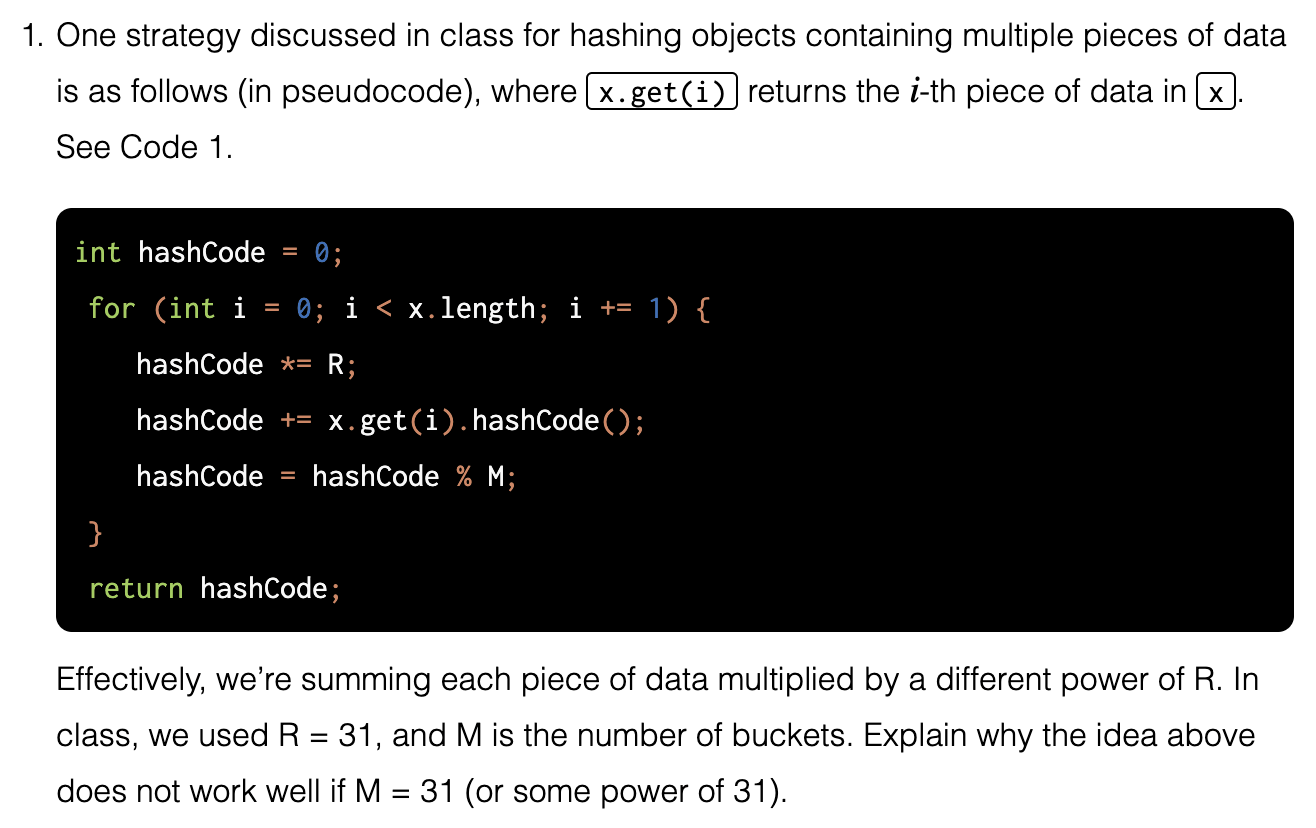For example: x is a - b - c

The 1st round:
hashCode = hc(a) % 31

The 2nd round:
hashCode = [(hc(a) % 31) * 31 + hc(b)] % 31 = hc(b) % 31

The 3rd round:
hashCode = hc(c) % 31

Problem: Only the last char matters here.

So finally, the result is hc(c) % 31. The first two items won’t play a role in hashing.

Also explain why the approach in A-level question 1 works better if we initially start the hashCode at 1 instead of 0.

// ——- To be done ——-
???
// ———————————

### Ex 3 (Same Hash Code)

Find the string with the same hash code in Java String.

A: 65
B: 66
a: 97 = B + 31

Aa: 65 31 + 97
AB: 66
31 + 66

How to generate strings that share the same hashcode in Java?

### Ex 4 (Permutation)

[Adapted from textbook 3.4.23] Suppose that we hash strings as described in A-level problem 1, using R = 256 and M = 255. Show that any permutation of letters within a string hashes to the same value. Why is this a bad thing?

Let the string be ab.

In the 1st round: $(a)\ \% \ 255$
In the 2nd round: $\left[(a)\ \% \ \times 256 + (b)\right]\ \%\ 255= \left[(a)\ \%\ 255 + ((a)\ \%\ 255) \times 255 + (b)\right] \ \%\ 255 = \left[ (a) + (b) \right]\ \%\ 255$

So, for ba, the hash code will be the same $\left[ (b) + (a) \right]\ \%\ 255$

### Ex 5 (Why start with 1)

In the 1st round:

• If hashCode starts at 0, $31 \times (a)\ \%\ 31 = 0$
• If hashCode starts at 1, $\left[31 + 31 \times (a)\right]\ \%\ 31 =$

### Ex 6 (2-Digit Hashing)

CS61B Fall 2009 midterm, #4 (really beautiful problem)

a)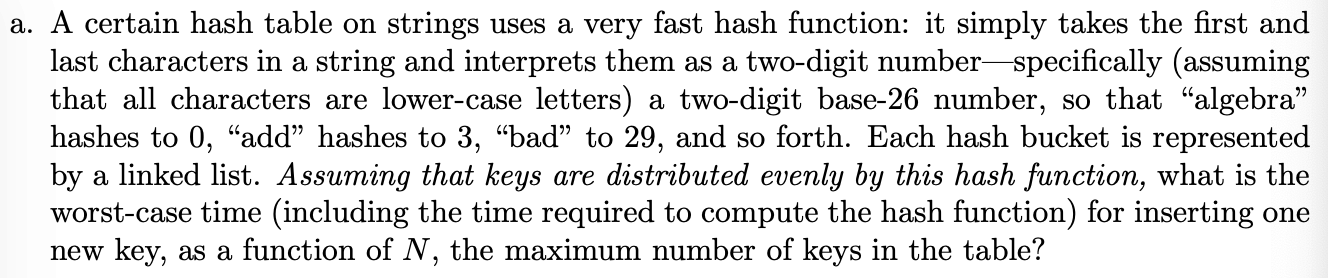Two-digit base-26 number means $26 \times (first) + (last)$.

algebra: 0 x 26 + 0 = 0
add: 0 x 26 + 3 = 3
bad: 1 x 26 + 3 = 29

There are $26^{2}$ combinations, so this is the number of buckets in total.

Since the hash function will divide the data evenly into only $26^2 = 676$ buckets, the time to check the selected bucket grows as $\frac{N}{676}$ at most.

Worst case runtime is $\Theta(N)$.

b) How does your answer to (a) change if we change the hash function so that it considers up to the first $\log_{26}{N}$ characters (or the length of the key, whichever is smaller), keeping other assumptions the same? (Ignore the time to compute $\log_{26}{N}$)

c) A heap data structure containing integers is represented as an array. Assuming that the heap is initially empty, show that for any $N$, there is a sequence of distinct $N$ values that can be added to the heap in total time $O(N)$.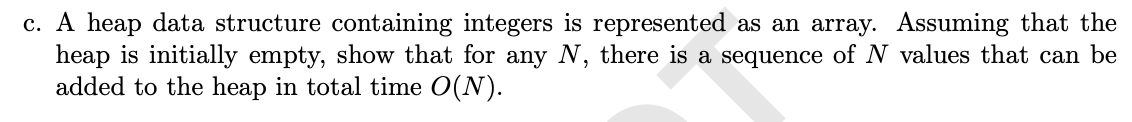d) For Java int variable $x$, what is (~x) - ((-1) ^ x)?

^ xor operator:

(~x) => 1 to 0, 0 to 1.

(-1) ^ x => 1 to 0, 0 to 1.

Answer: 0.

## HW 3: Oomage Hash

HW 3: link
Code: link

### equals

How to write equals method?

equals method should have the following properties to be in compliance:l

• Reflexive: x.equals(x) must be true for any non-null x.
• Symmetric: x.equals(y) must be the same as y.equals(x) for any non-null x and y.
• Transitive: if x.equals(y) and y.equals(z), then x.equals(z) for any non-null x, y, and z.
• Consistent: x.equals(y) must return the same result if called multiple times, so long as the object referenced by x and y do not change.
• Not-equal-to-null: x.equals(null) should be false for any non-null x.

### Modulus

For the purposes of converting an Oomage’s hashCode to a bucket number, you should compute bucketNum = (o.hashCode() & 0x7FFFFFFF) % M, where o is an Oomage. You should not use Math.abs or Math.floorMod.

Math.abs:

1111 1111 => 255
1111 1111 => -1

If you do Math.abs(-2147483648), it will still output -2147483648 since -(-2147483648) = 2147483648 overflows.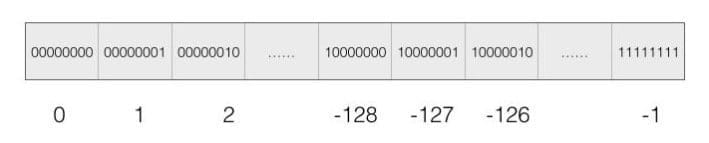Just like 127 + 1 => -128.

## Lab 8: HashMap

Lab 8 in Spring 2019 (similar to Lab 9 in Spring 2018).

My code: link

Algs4 code: link

## Priority Queue

### Interface

Usage Example: Unharmonious Texts

• Your job: Monitor the text messages of the citizens to make sure that they are not having any unharmonious conversations.
• Each day, you prepare a report of the M messages that seem most unharmonious using the HarmoniousnessComparator.

Naive Approach: Create a list of all messages sent for the entire day. Sort it using your comparator. Return the M messages that are largest.

Better Implementation: Track the M Best

How Would We Implement a MinPQ?

Some possibilities:

• Ordered Array
• Bushy BST: Maintaining bushiness is annoying. Handling duplicate priorities is awkward.
• HashTable: No good! Items go into random places.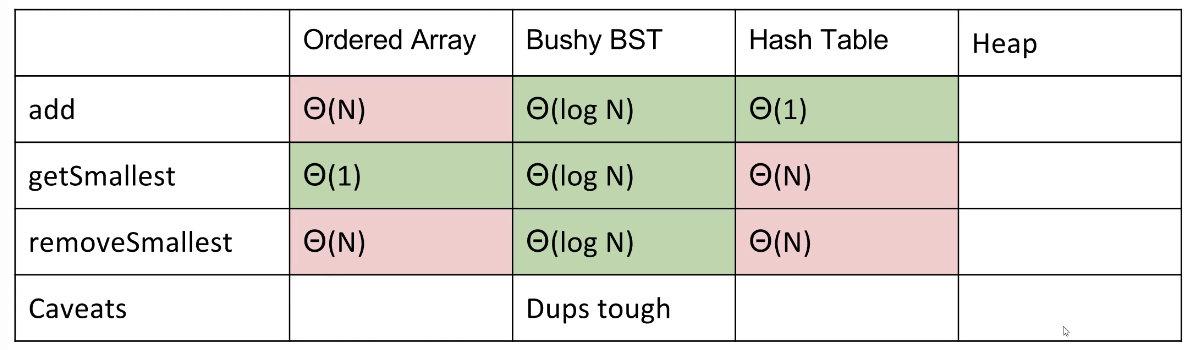### Tree Representations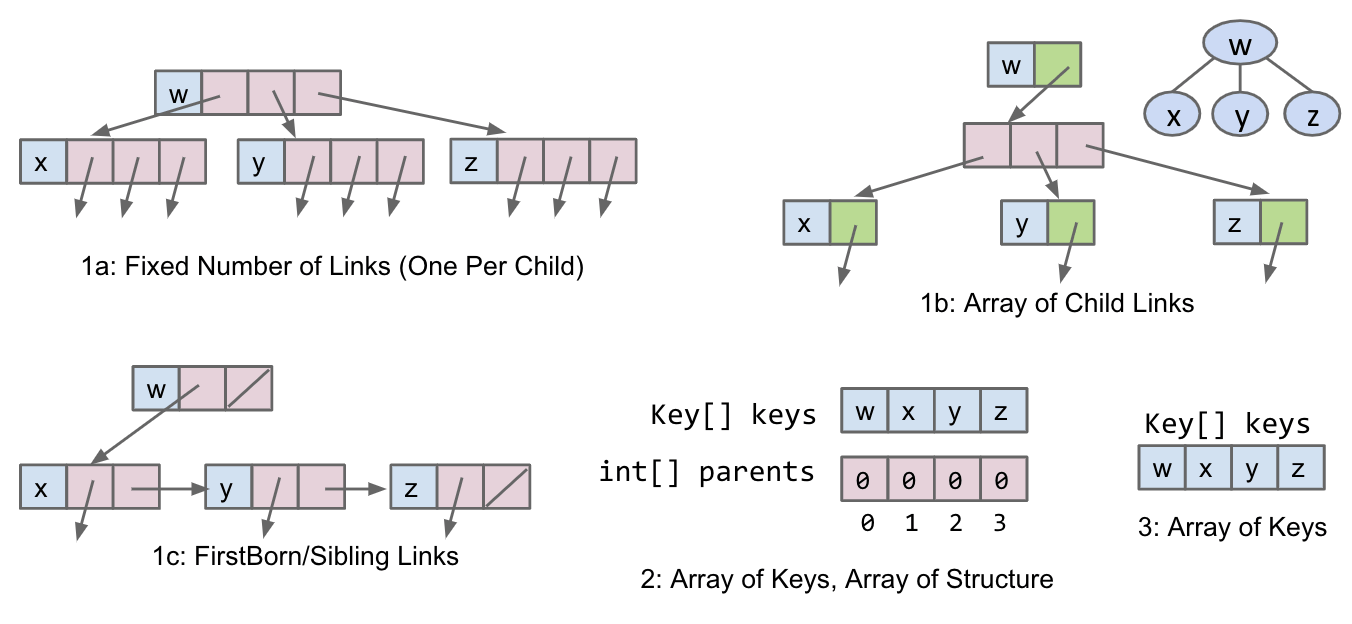#### Approach 1

Approach 1A

Consider creating pointers to our children and storing the value inside of the node object. It gives us fixed-width nodes.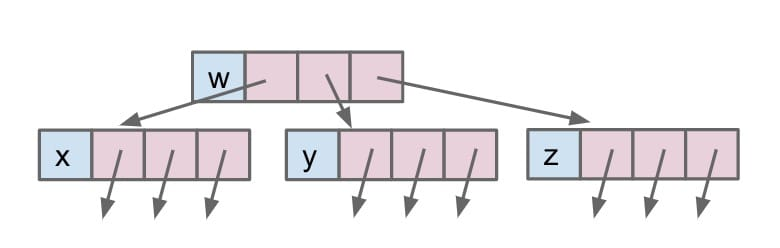Approach 1B

We explore the use of arrays as representing the mapping between children and nodes. This would give us variable-width nodes, but also awkward traversals and performance will be worse.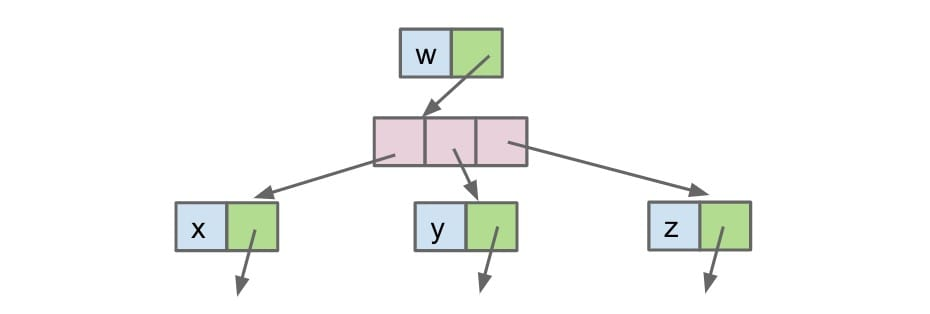Approach 1C

Lastly, we can use the approach for Tree1C. This will be slightly different from the usual approaches that we’ve seen. Instead of only representing a node’s children, we say that nodes can also maintain a reference to their siblings.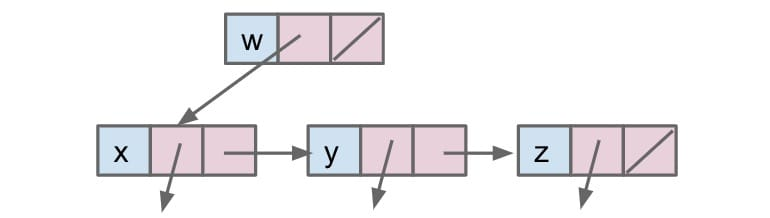In summary, in the 1st approach we store explicit references to who is below us (actual tree children objects).

#### Approach 2

Without explicit references to children objects.

Recall the Disjoint Sets ADT. The way that we represented this Weighted Quick Union structure was through arrays. For representing a tree, we can store the keys array as well as a parents array.

• The keys array represents which index maps to which key.
• The parents array represents which key is a child of another key.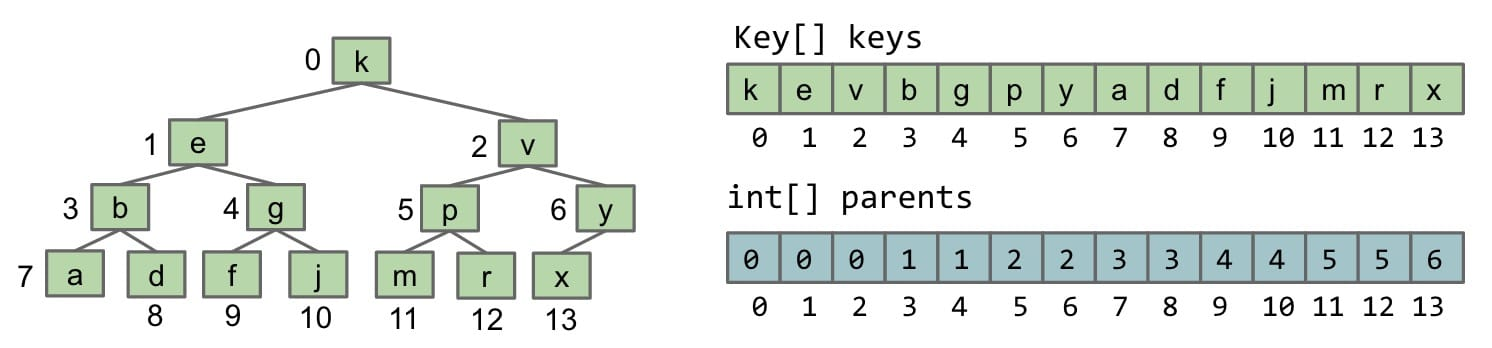parent = (child - 1) / 2

It’s time to make a very important observation! Based on the structure of the tree and the relationship between the array representations and the diagram of the tree, we can see:

• The tree is complete. This is a property we have defined earlier.
• The parents array has a sort of redundant pattern where elements are just doubled.
• Reading the level-order of the tree, we see that it matches exactly the order of the keys in the keys array.

#### Approach 3

In this approach, we assume that our tree is complete. This is to ensure that there are no gaps inside of our array representation. So we can flatten this complex 2D structure of the tree and flatten it into an array.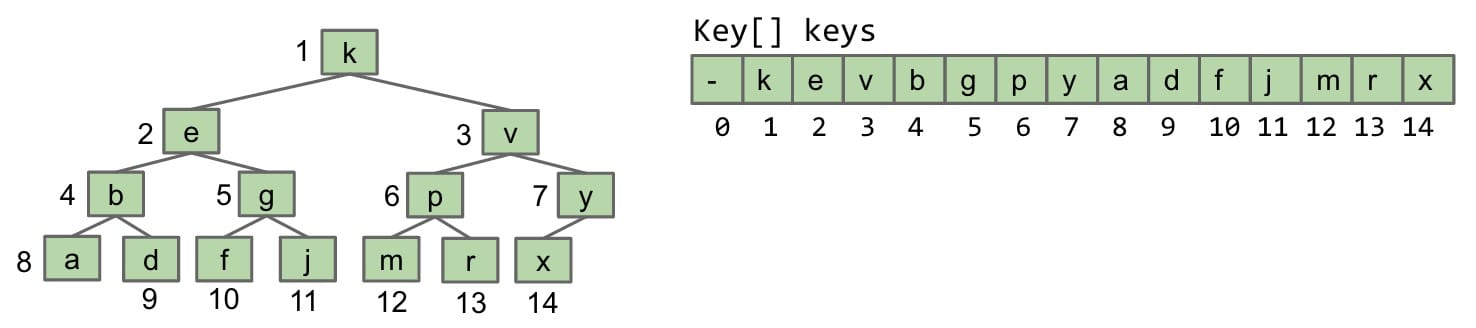This is the underlying implementation for the Priority Queue ADT.

How to get a node’s parent?

How to get a node’s left or right child?

Swim code:

### Heaps

BSTs would work, but need to be kept bushy and duplicates are awkward.

Binary min-heap: Binary tree that is complete and obeys min-heap property.

• Complete: Missing items only at the bottom level (if any), all nodes are as far left as possible.
• Min-heap: Every node is less than or equal to both of its children.#### Construction

• Heap Construction (Heapification): Sink or swim items in array (Usually when we update an item’s key) => $O(N\log{N})$
• The order of sink() or swim() doesn’t matter.
• Quicksort: Partition once on every item (pivot) => $O(N\log{N} + N)= O(N\log{N})$
• MinHeap: Add in increasing order.
• Up-Bottom Heapification: The following contents from 61B are based on this approach. => $O(N\log{N})$
• Insert an item into an array from the left side, or building from the top of the heap. (Place the item at current rightmost position)
• Swim the inserted item if necessary.
• Bottom-up Heapification: A better approach (will explain). => $O(N)$
• Insert an item into an array from the right side, or building from the bottom of the heap.
• Sink the inserted item if necessary.

Why?

Sink is better than Swim. Assuming a heap is of height $\log{N}$, sinking from the top takes $1 \times \log{N}$ at most; while swimming from the bottom (when it is full of nodes) takes $N / 2 \times \log{N}$.

Swim (Up-Bottom):

Sink (Bottom-Up):

The Up-Bottom Heapification is presented by 61B in the next section.

Here is the process of Bottom-Up heap construction.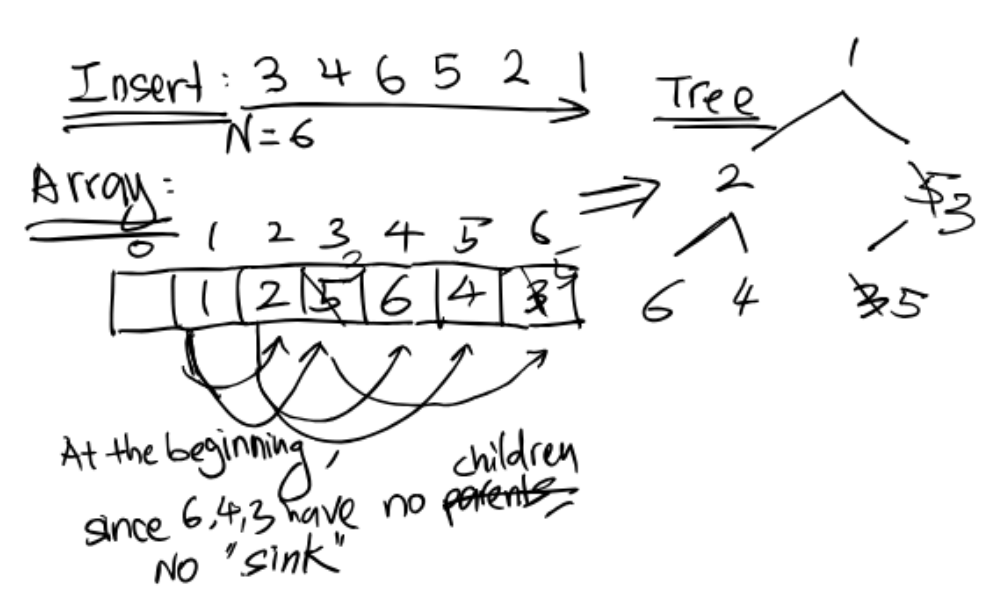#### Operations

Add: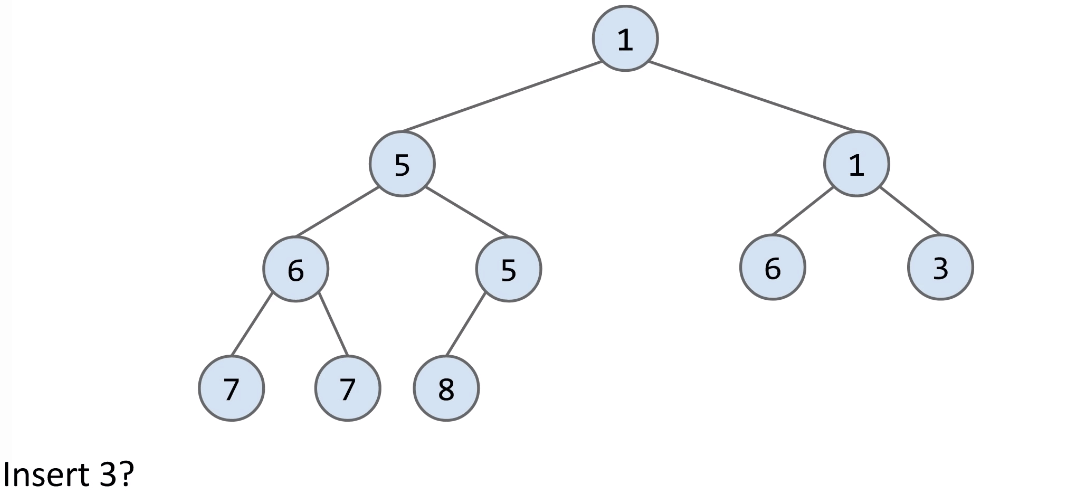• Add to end of heap temporarily.
• Swim up the hierarchy to your rightful place.

Delete: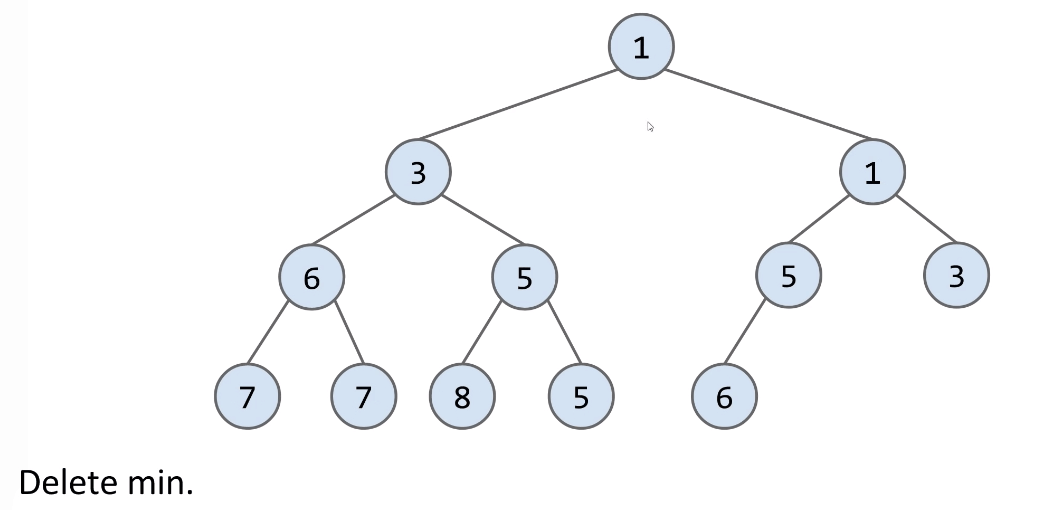• Swap the last item in the heap into the root.
• Then sink your way down the hierarchy, yielding to most qualified folks.

Summary

• getSmallest(): Return the item in the root node.
• add(x): Place the new employee in the last position, and promotes as high as possible.
• removeSmallest():
• Assassinate the president (of the company), promote the rightmost person in the company to president. Then demote repeatedly, always taking the ‘better’ successor.
• Or, swap the last item in the heap into the root. Sink down the hierarchy to the proper place. Sinking involves swapping nodes if parent > child. Swap the smallest child to preserve min-heap property.
• How could we get the last item => How to better represent a tree?

#### Implementation

##### Approach 1

MyHeap.java (very interesting!)

It turns out that I did extra comparisons, but it worthed trying.

1) Use helper methods like size() & height()

With this code, we don’t write:

Instead, we should write:

2) See promote() & demote()

promote():

Why this swapping works?

If I swap(B,C), will C be greater than A?

No. B < A. After swap(B,C), C < B. So C < B < A and C is the parent.

Also, a better way to think about it is to make the tree a list.

Actually, it is too much. It could be simpler.

When the node is being promoted, we just need to compare the node to its parent, not its sibling.

Because A < B, after swap(A,C) C is less than B.

However, the data structure determines I can only compare twice because I can’t look to a node x‘s parent. If x is the parent, I don’t know which child should be compared with.

demote():

See the comment.

##### Approach 3B

My HeapPQ Implementation (Based on Approach 3B): (https://github.com/junhaowww/cs61b-sp18/blob/master/clab8/HeapPQ.java)

It allows comparators to support MinPQ and MaxPQ. It includes testing.

#### Runtime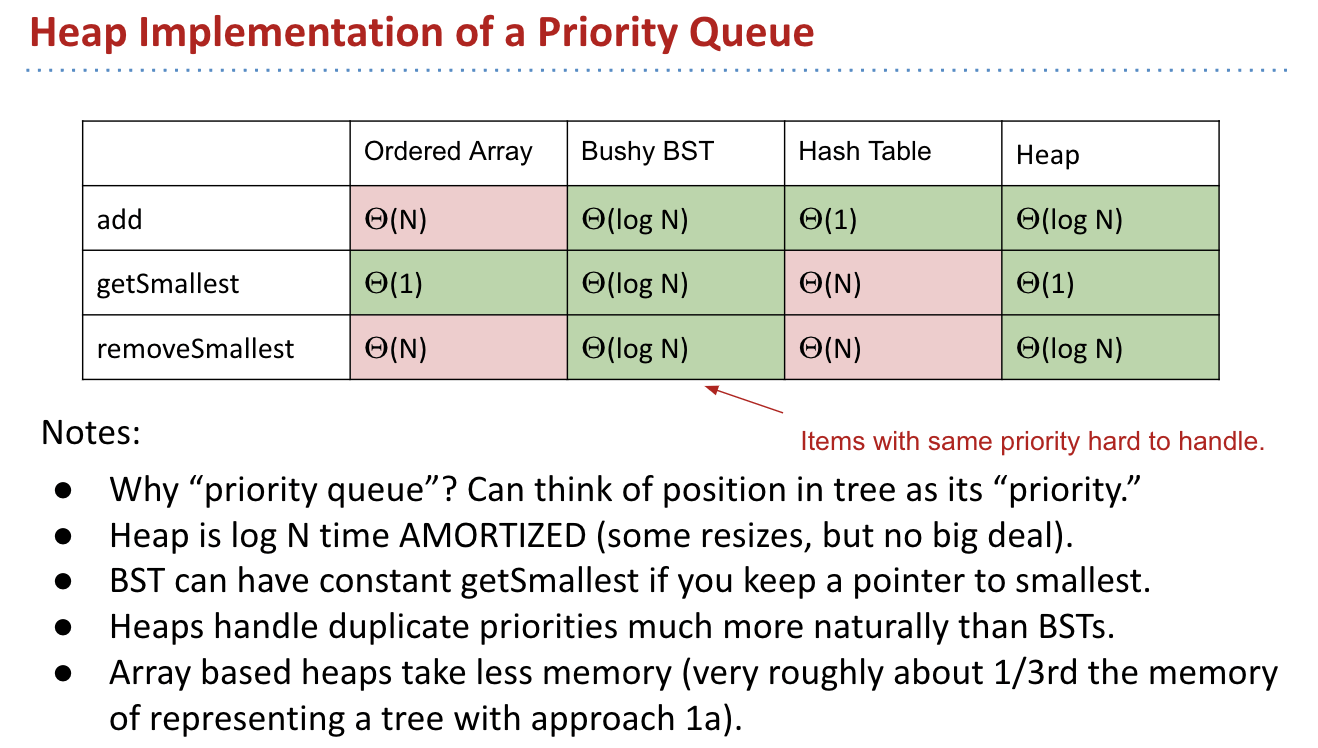## Ex (Discussion)

### Ex 1 (Heaps of Fun)

Just simple practice.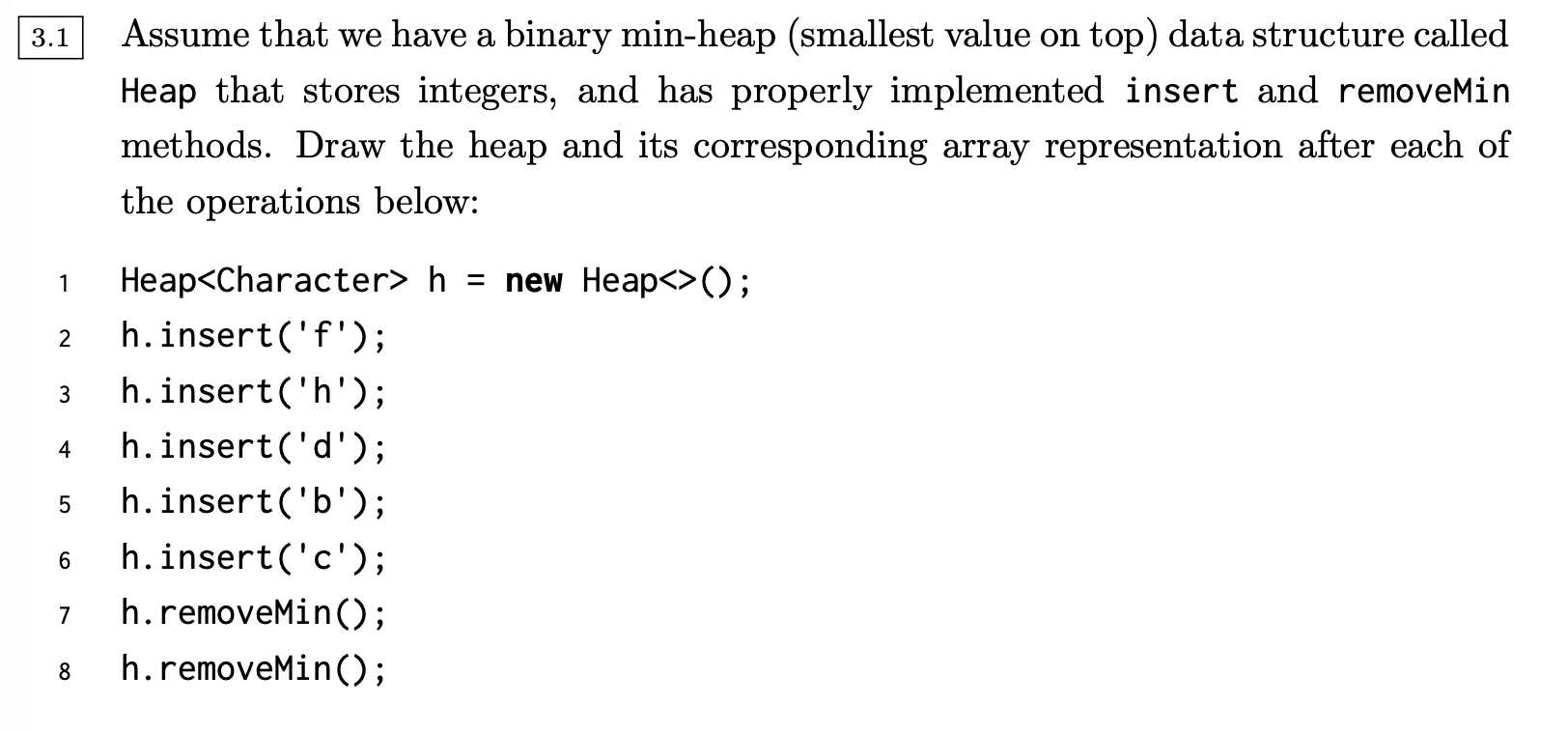Answer: my drawing

## Ex (Examprep)

### Ex 1 (Median Value)

Consider the min heap below , where each letter represents some value in the tree. Assume each value in the tree is unique.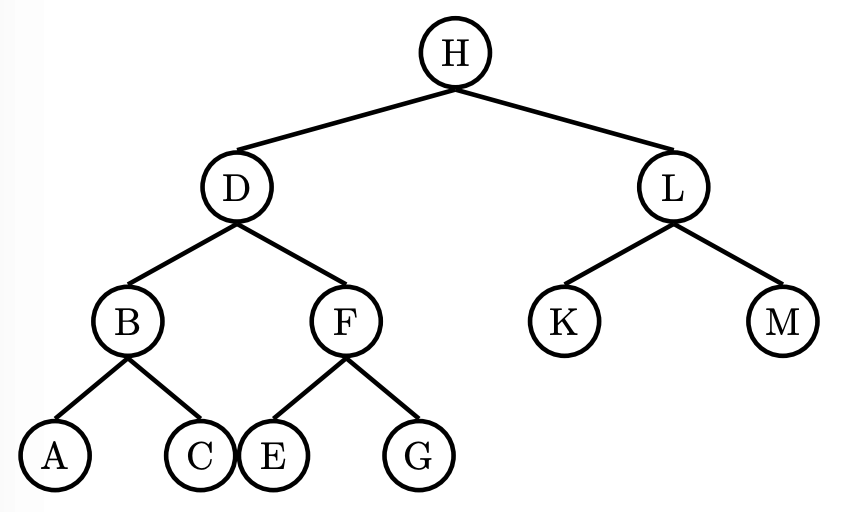(a) Assuming values are inserted into the heap in increasing order, indicate all letters which could represent the following values:

Smallest value: H
Median value: K
Largest value: G

(b) Assuming values are inserted into the heap in decreasing order, indicate all letters which could represent the following values: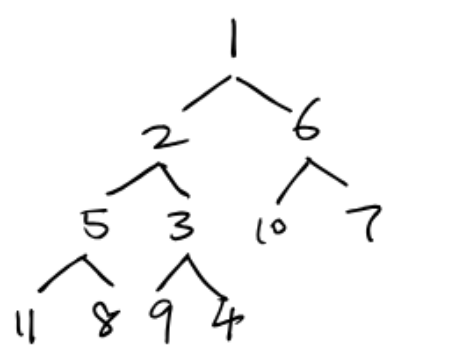Smallest value: H
Median value: L
Largest value: A

(c) Assuming values are inserted into the heap in an unknown order, indicate all letters which could represent the following values:

As for median:

1st: Impossible.
2nd: Only L

If D is the median, it can hold 6 elements larger or equal than itself, but there are only 5 elements.

3rd layers: B, F, K, M
4th layers: A, C, E, G

Smallest value: H
Median value: L, B, F, K, M, A, C, E, G
Largest value: A, C, E, G

## Ex (Guide)

Priority Queue. A Max Priority Queue (or PQ for short) is an ADT that supports at least the insert and delete-max operations. A MinPQ supports insert and delete-min.

Heaps. A max (min) heap is an array representation of a binary tree such that every node is larger (smaller) than all of its children. This definition naturally applies recursively, i.e. a heap of height 5 is composed of two heaps of height 4 plus a parent.

### C level

#### Ex 1 (Add Order)

Is an array that is sorted in descending order also a max-oriented heap?

Yes.

#### Ex 2 (Easy)

Problem 3 from Princeton’s Fall 2009 midterm: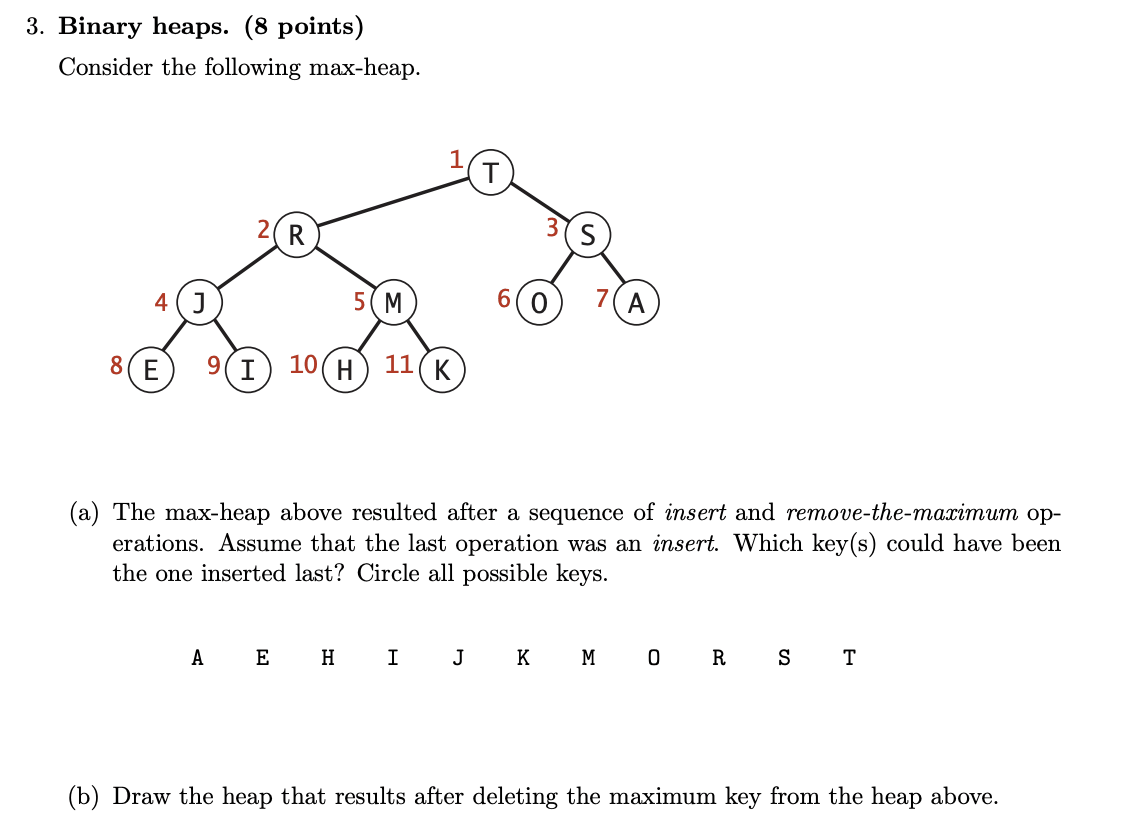(a) Along the swimming (promoting) path: K, M, R, T.

(b)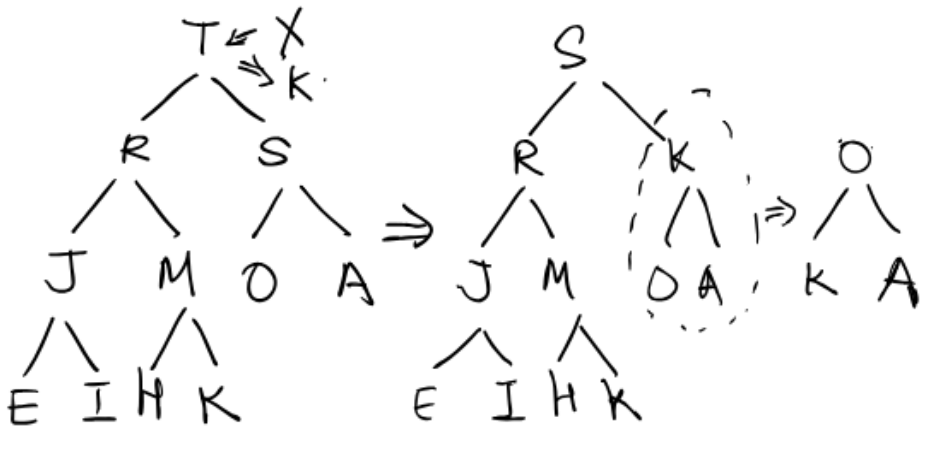#### Ex 3 (Change or Not?)

Problem 4 from Princeton’s Fall 2008 midterm:

(c) True or false: given any binary heap with $N$ distinct keys, the result of inserting a key larger than any of the $N$ keys, and then deleting the maximum key yields the original binary heap.

True. The same path when swimming and sinking.

### B level

#### Ex 1 (The k-th largest)

(Textbook 2.4.7) The largest item in a heap must appear in position 1, and the second largest must appear in position 2 or 3. Give the list of positions in a heap of size 31 where the k-th largest CAN appear, and where the k-th largest CANNOT appear for k = 2, 3, 4. Assume values are distinct.

k = 2: 2nd or 3rd
k = 3: 2nd or 3rd or 4th ~ 7th
k = 4: 2nd or 3rd or 4th ~ 7th or 8th ~ 15th

#### Ex 2 (Stack and Queue with PQs)

(Textbook 2.4.21) Explain how to use a priority queue to implement the stack and queue data types.

Interesting!

• Stack: FILO

For example, we have a min-heap. To guarantee getSmallest, we have to add in decreasing order (MAX -> MIN).

So we need another max-heap and add all values into the max-heap first, and then get the maximum and put it into the min-heap.

• Queue: FIFO

For example, we have a min-heap. To guarantee getSmallest, we have to add in decreasing order (MIN -> MAX).

So we need another min-heap.

#### Ex 3 (getMin in MaxPQ)

(Adapted from Textbook 2.4.27). Add a min() method to a maximum-oriented PQ. Your implementation should use constant time and constant extra space.

Interesting!

Need one field to record the minimum value and initialize it as minus infinity. Each time a new item is added we need to make a comparison and update the minimum value if necessary.

Besides, we don’t need to make a big change in the getLargest (assume it includes removing) method because it works on the largest number which has no effect on the smallest one except there is only one item.

#### Ex 4 (#Exchange)

(Textbook 2.4.14) What is the minimum number of items that must be exchanged during a remove-the-max operation in a heap of size $N$ with no duplicate keys? Give a heap of size 15 for which the minimum is achieved. Answer the same question for two and three successive remove-the-maximum operations.

For size = 15, the minimum number of exchanging (not including replacing the root) is 1 if size > 3.

The last item could be demoted to the left or right.

Visualization:

For example, we are demoting 1. What if the item at  (14 is just an example) is very small. So 1 has to go to left. Then it may stop or continue demoting.

It depends on the values like , , , .

Not finished.

#### Ex 5 (k-heap)

Problem 4 from Princeton’s Spring 2008 midterm.

Interesting!

(a) What does it output? It outputs the k smallest Integers in descending order.

(b) What is the order of growth of its worst-case running time.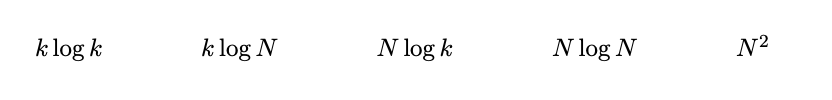insert: N (a[] is in decreasing order) -> $N\log{N}$

Note: The maximum size of the heap is $k$.

insert: $N \log{k}$
MARK: $(N - k) \times \log{k}$
println: $k \log{k}$

Total: $N\log{k} + (N - k) \times \log{k} + k \log{k} = N \log{k}$

If the marked line was deleted. Repeat the two questions.

(c) What does it output? It outputs the k largest Integers in descending order.

(d) What is the order of growth of its worst-case running time.

Note: The maximum size of the heap is $N$.

insert: $N \log{N}$
println: $k \log{N}$

Total: $N\log{N} + k \log{N} = N\log{N}$

($N\log{N} + k\log{N} \leq N\log{N} + N\log{N} = 2N \log{N} \sim N\log{N}$)

#### Ex 6 (Zork Sort)

Problem 6 from Hug’s Fall 2014 midterm.

Suppose you are given an array A with $n$ elements such that no element is more than $k$ slots away from its position in the sorted array. Assume that $k > 0$ and that $k$, while not constant, is much less than $n$ ($k << n$).

If k = 1, it is sorted.

a) Fill in the blanks such that array A is sorted after execution of this method. The important operations on a PriorityQueue are add(x), remove(), and isEmpty(). Your solution should be as fast as possible.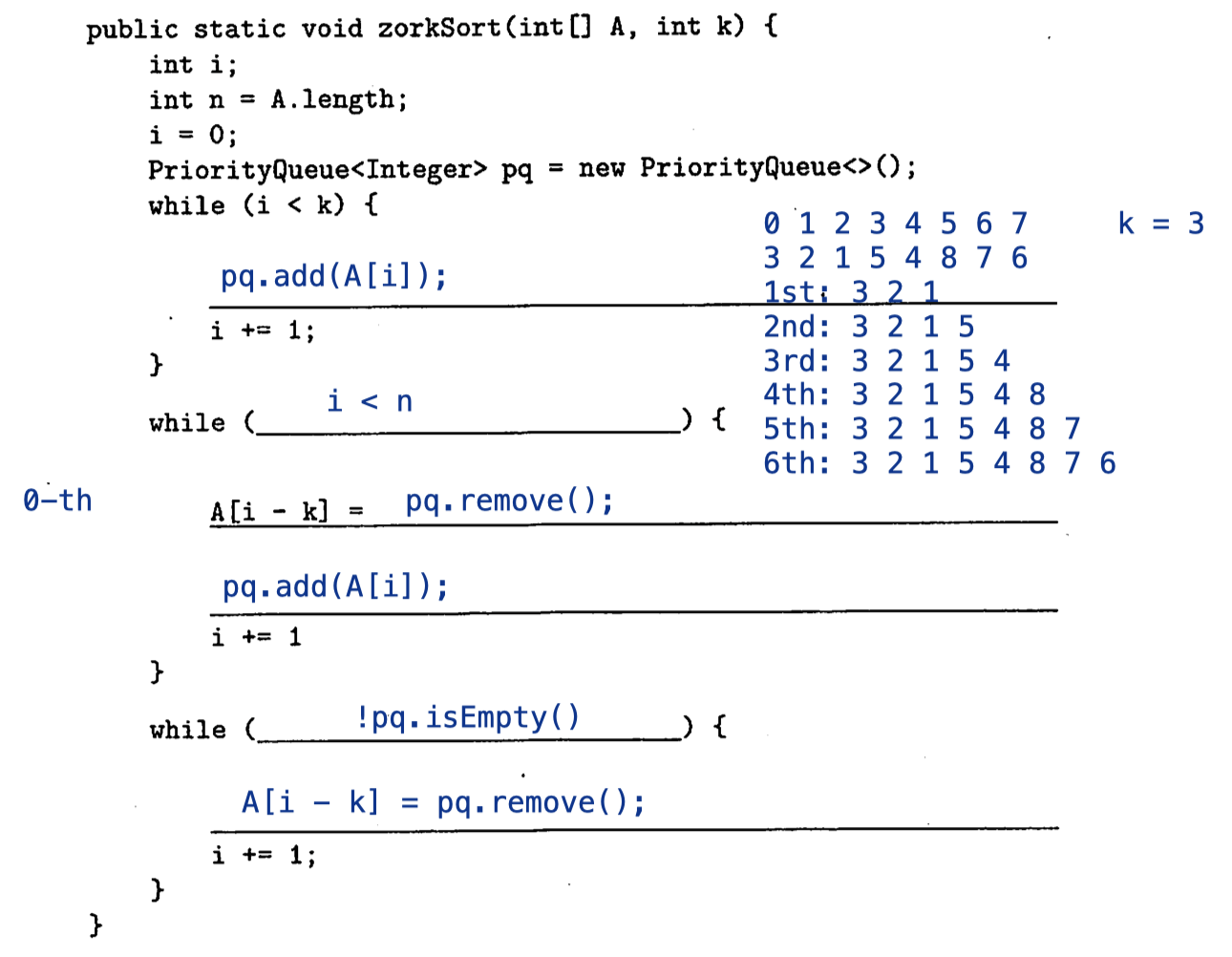b) What is the running time of this algorithm, as a function of $n$ and $k$? Justify you answer.

$O(n\log{k})$

Go over approximately $n$ elements and each time remove or add takes $\log{k}$ since the size of the PriorityQueue is $k$.

#### Ex 7 (3-heap)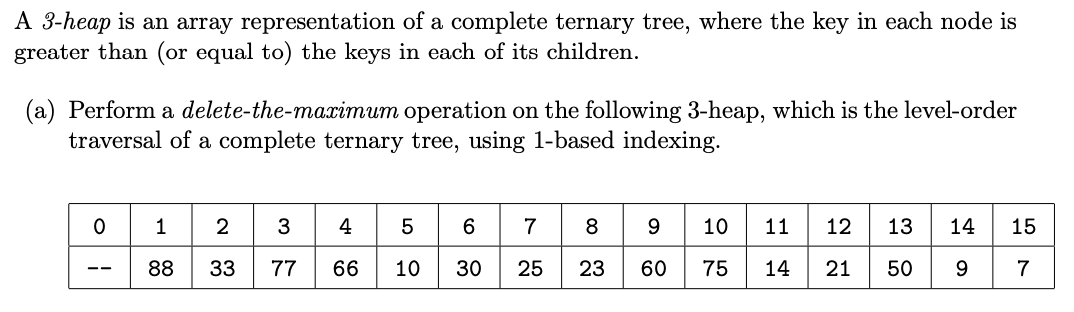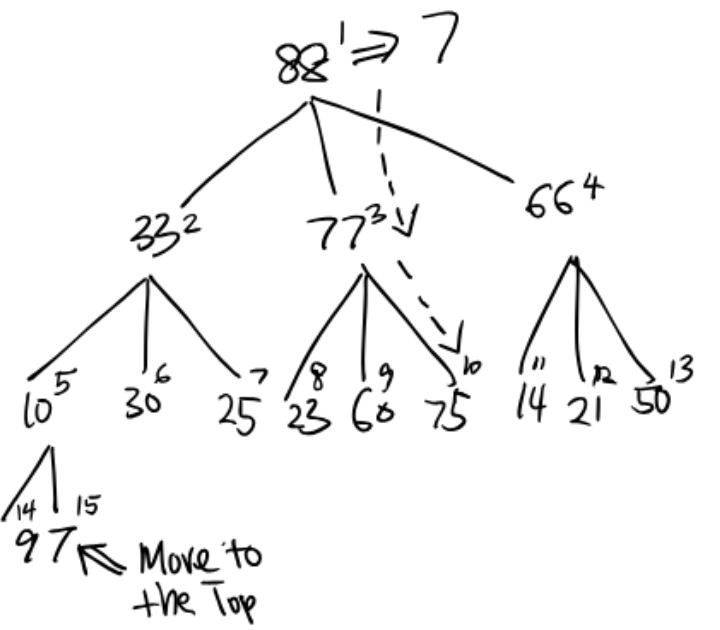(b) Given the array index $k$ of a key, what are the indices of its three (potential) children as a function of $k$? Assume 1-based indexing.

For parent $k$ and child $p$:

• Left child = $3k - 1$
• Middle child = $3k$
• Right child = $3k + 1$
• Parent = $\frac{p + 1}{3}$

(c) What is the maximum number of compares for a delete-the-maximum operation as a function of the number of keys $N$ in the data structure?

To sink a key, we first find the largest child and also determine if it is less than the largest child.

The first step takes 2 compares and the second step takes only 1 compare. So in total it takes 3 compares in each level.

Since the height of a 3-heap is $\sim \log_3{N}$, the total number of compares in the worst case is $\sim 3\log_3{N}$.

#### Ex 8 (Bottom-Up Heapification)

Problem 5 from Hug’s Spring 2013 midterm.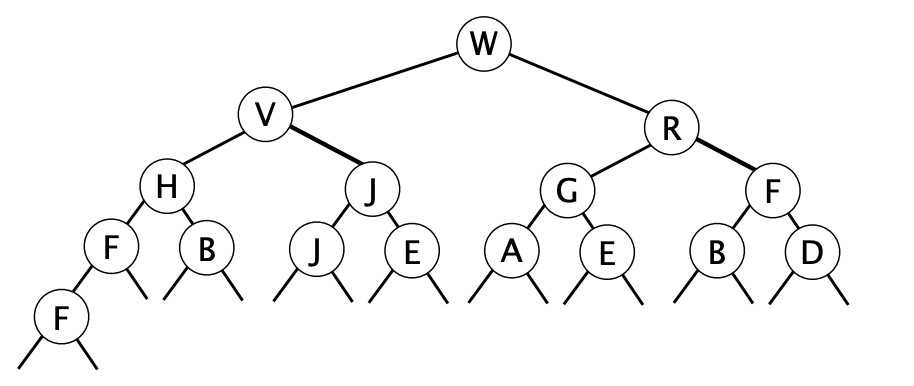(b) Consider the process of creating a max heap from an arbitrary text file containing $N$ integers. What is the order of growth of the run time of the best possible algorithm for this process?

Heapification is linear time $O(N)$. Best algorithm is to use bottom-up heapification or bottom-up heap construction, where each node is successively sunk starting with the rightmost element of the array and working backwards to the left. (versus the Up-Bottom Heapification presented by 61B which takes $O(N\log{N})$)

(c) Is there any reason in terms of time or space efficiency to use a max heap to implement a max priority queue instead of using an LLRB tree? Justify your answer.

Linearithmic: Taking up to time proportional to $N\log{N}$ to run on inputs of size $N$.

• Time saving. Bottom-up heapification is linear time $O(N)$, whereas constructing an LLRB takes linearithmic time. Finding the max in a max heap is constant time $O(1)$, but in an LLRB it is logarithmic time $O(\log{N})$.
• Space saving. A max heap uses less memory since we don’t need to store lots of node objects including links and colors.

### A level

#### Ex 1 (Median PQ)

Design a data type that supports insert in $O(\log{N})$ time, find-the-median in $O(1)$ time, and delete-the-median in $O(\log{N})$ time.

Super interesting! I like this one!

Use 2 PQs: maxPQ and minPQ. Left-side values are stored in maxPQ, while the right-side values are stored in minPQ.

#### Ex 2 (Max-Min PQ)

Design a data type that supports insert in $O(\log{N})$ time, delete-the-max in $O(\log{N})$ time, and delete-the-minimum in $O(\log{N})$ time.

Again. Interesting!

Possible solution: minPQ (maxMid) + (minMid) maxPQ

But actually remove may cause bad things to occur. What happens if I delete all from minPQ leaving maxPQ with many items?

The simple way is to just use a binary search tree, as M. Shaw and user2357112 recommend.

Two Binary Heaps

If you’re required to build this on top of binary heaps, then in each heap, alongside each element, store the element’s position in the other heap. Every time you move an element in one heap, you can go straight to its position in the other heap and update it. When you perform a delete-min or delete-max, no expensive linear scan in the other heap is required.

Or use hash table to index, as Eric Z mentions: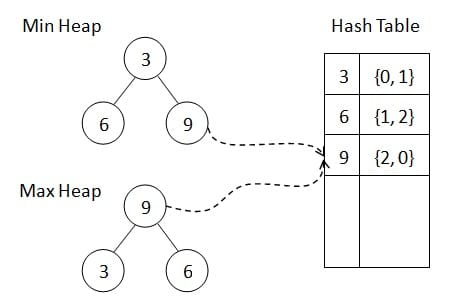#### Ex 3 (Stable PQ)

Problem 7 from Princeton’s Fall 2010 midterm.

A min-based priority queue is stable if min() and deleteMin() return the minimum key that was least-recently inserted. Describe how to implement a StableMinPQ data type such that every operation takes at most (amortized) logarithmic time.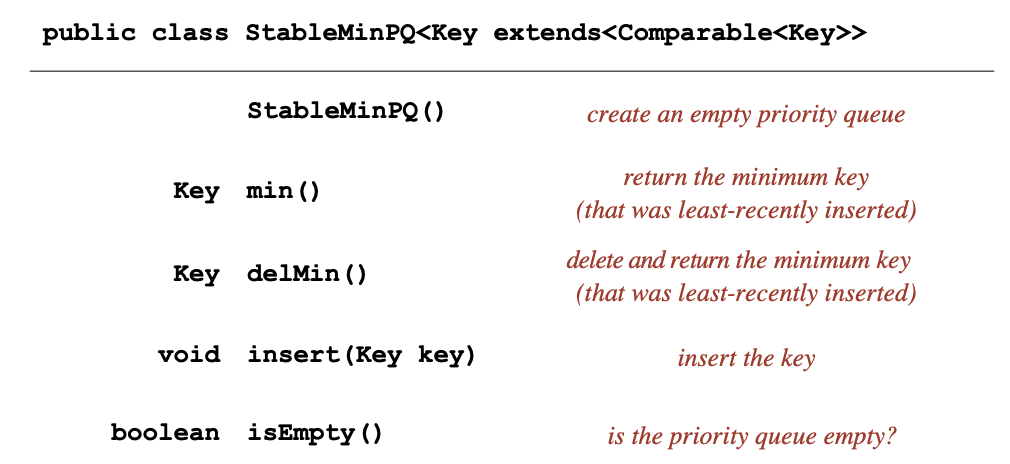Reference: Solution from Princeton

Binary heap solution:

The basic idea is to associate the integer timestamp $i$ with the $i^{th}$ key that is inserted. To compare two keys, compare their keys as usual; if they are not equal, break ties according to the associated timestamp. This ensures that if there are two equal keys, the one that was inserted first is considered smaller than the one that was inserted last. (Example: Stable Priority Queues?)

Or by adding a parallel array long[] timestamp as an instance variable of StableMinPQ.

Binary search tree solution:

To handle duplicate keys, we declare a RedBlackBST<Key, Queue<Key>>, where the value is a queue of all the keys equal to the key.

• To insert a key, we check if there is a key equal to it already in the BST. If there is, we add the key to the corresponding queue.
• To delete the minimum key, we call min() to find the minimum, and return the first element of corresponding queue.

#### Ex 4 (Random PQ)

Problem 8 from Princeton’s Spring 2012 midterm.

Describe how to add the methods sample() and delRandom() to our binary heap implementation of the MinPQ API. The two methods return a key that iss chosen uniformly at random among the remaining keys, with the latter method also removing that key.

You should implement the sample() method in constant time and the delRandom() method in time proportional to $\log{N}$, where $N$ is the number of keys in the data structure. For simplicity, do not worry about resizing the underlying array.

sample(): Select a random array index $r$ (between $1$ and $N$) and return the key $a[r]$.

delRandom():

• Select: Do sample() and save away the key $a[r]$, to be returned.
• Delete: Swap $a[r]$ and $a[N]$ and decrement $N$.
• Restore: Restore heap order invariants.

• Call sink(r) if $a[N]$ was too big.
• Call swim(r) if $a[N]$ was too small. (Since $a[N]$ might not be the largest key)
• Note: Although two methods are called, the actual swapping would only happen at one direction (up or down) or no swapping happens if $a[N]$ fits quite well.

• Note: The order of sink and swim does not matter.

Note: sample() and delRandom() may not refer to the same key. One way to achieve is to add a field randomIndex and additional helper method random() to generate the randomized index. Then both two methods above refer to this index.

0%